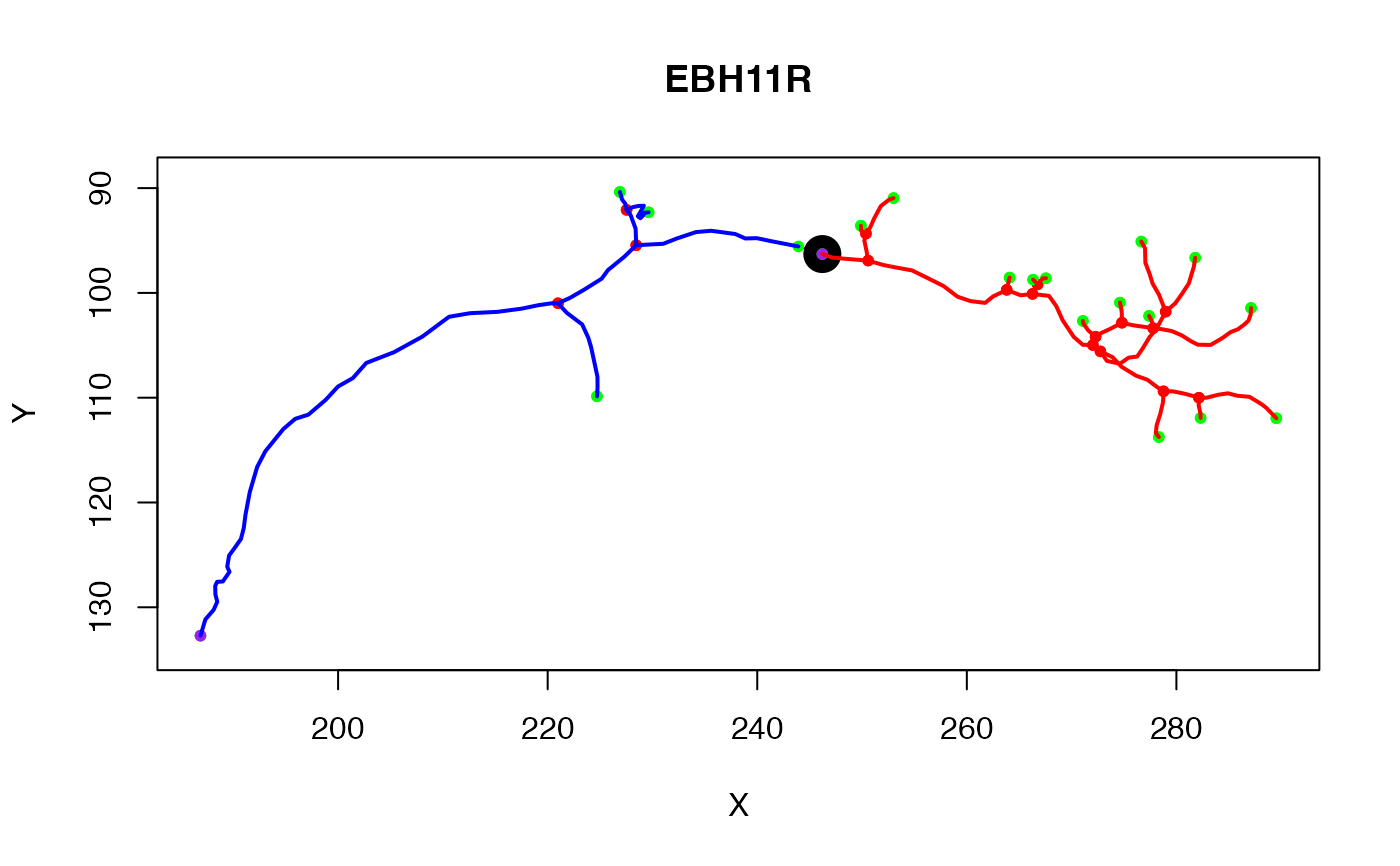Subset neuron by keeping only vertices that match given conditions

# S3 method for neuron
subset(x, subset, invert = FALSE, ...)

## Arguments

x

A neuron object

subset

A subset of points defined by indices, an expression, or a function (see Details)

invert

Whether to invert the subset criteria - a convenience when selecting by function or indices.

...

Additional parameters (passed on to prune_vertices)

subsetted neuron

## Details

subset defines which vertices of the neuron to keep and is one of

• logical or numeric indices, in which case these are simply used to index the vertices in the order of the data.frame x$d. Note that any NA values are ignored. • a function (which is called with the 3D points array and returns T/F vector) • an expression evaluated in the context of the x$d data.frame containing the SWC specification of the points and connectivity of the neuron. This can therefore refer e.g. to the X,Y,Z location of vertices in the neuron.

Note that due to its use of non-standard evaluation subset.neuron, which is convenient interactive use but can be fragile when used inside other functions. If you run into trouble it is recommended to use the underlying prune_vertices function.

prune.neuron, prune_vertices, subset.dotprops

Other neuron: neuron(), ngraph(), plot.dotprops(), potential_synapses(), prune(), resample(), rootpoints(), spine()

## Examples

n=Cell07PNs[]
# keep vertices if their X location is > 2000
n1=subset(n, X>200)
# diameter of neurite >1
n2=subset(n, W>1)
# first 50 nodes
n3=subset(n, 1:50)
# everything but first 50 nodes
n4=subset(n, 1:50, invert=TRUE)

## subset neuron by graph structure
# first plot neuron and show the point that we will use to divide the neuron
n=Cell07PNs[]
plot(n)
# this neuron has a tag defining a point at which the neuron enters a brain
# region (AxonLHEP = Axon Lateral Horn Entry Point)
points(t(xyzmatrix(n)[n$AxonLHEP, 1:2]), pch=19, cex=2.5) # now find the points downstream (distal) of that with respect to the root ng=as.ngraph(n) # use a depth first search distal_points=igraph::graph.dfs(ng, root=n$AxonLHEP, unreachable=FALSE,
neimode='out')\$order
distal_tree=subset(n, distal_points)

# Find proximal tree as well
# nb this does not include the AxonLHEP itself as defined here
proximal_points=setdiff(igraph::V(ng), distal_points)
proximal_tree=subset(n, proximal_points)if (FALSE) {
## subset using interactively defined spatial regions
plot3d(n)
# nb you can save this select3d object using save or saveRDS functions
# for future non-interactive use
s3d=select3d()
n4=subset(n, s3d(xyzmatrix(n)))
# special case of previous version
n5=subset(n, s3d)
stopifnot(all.equal(n4,n5))
# keep the points that were removed from n1
n4.not=subset(n,Negate(s3d))
# vertices with x position > 100 and inside the selector function
n6=subset(n,X>100 & s3d(X,Y,Z))

## subset each neuron object in a whole neuronlist
n10=Cell07PNs[1:10]
plot3d(n10, lwd=0.5, col='grey')
n10.crop = nlapply(n10, subset, X>250)
plot3d(n10.crop, col='red')

## subset a neuron using a surface
library(nat.flybrains)
# extract left lateral horn surface and convert to mesh3d
lh=as.mesh3d(subset(IS2NP.surf, "LH_L"))
# subset neuron with this surface
x=subset(Cell07PNs[], function(x) pointsinside(x, lh))